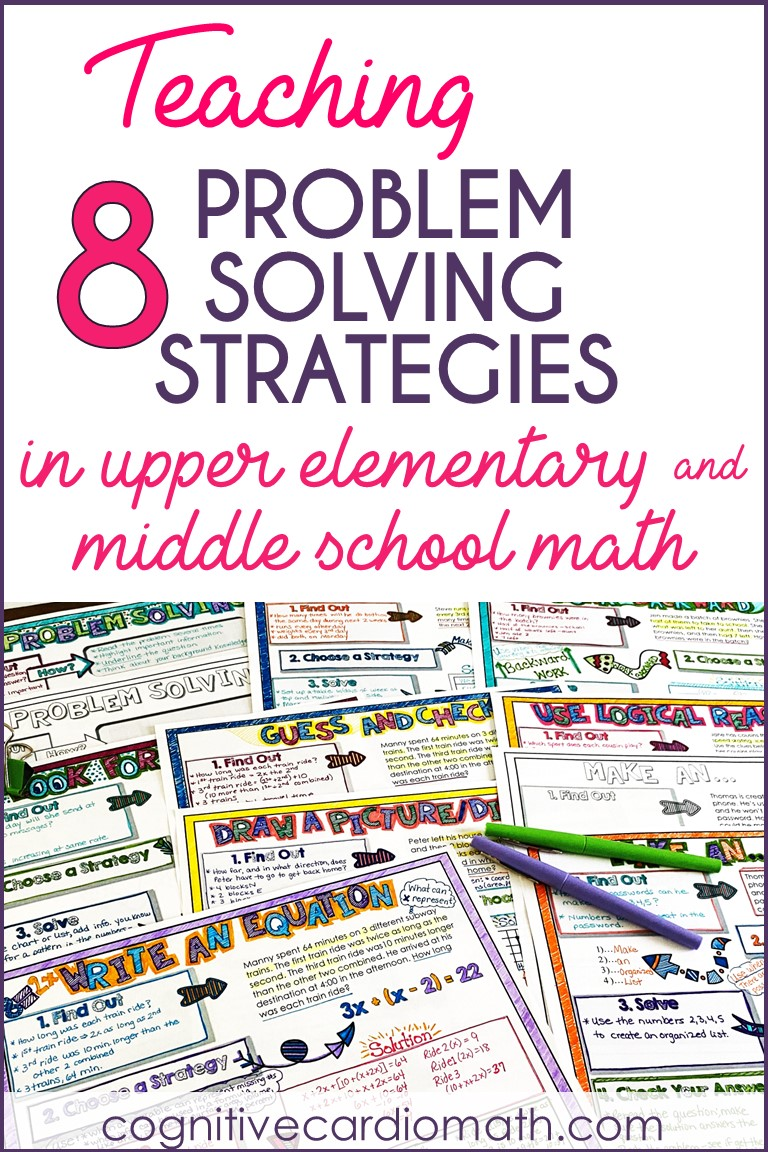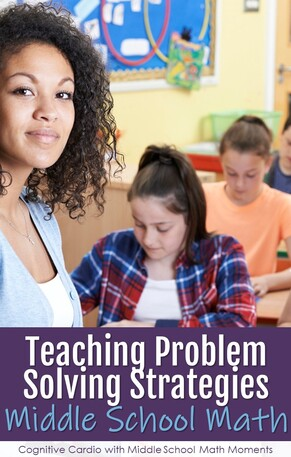# Teaching Math Problem Solving Strategies

## Teaching Math Problem Solving Strategies in Middle School

There are many math problem solving strategies out there and some are very beneficial to upper elementary and middle school math students.

Before we jump into them, I’ll share a little bit of my experiences in teaching math problem solving strategies over the past 25+ years.

During my second year of teaching (in the early 90s), I was teaching 5th grade, and our state math testing began to include a greater focus on problem solving and writing in math.Over the next several years, the other math teachers and I used standard sentence starters to help math students practice explaining their problem solving process. These were starters like:

• “In this problem, I need to….”
• “From the problem, I know….”
• “To solve the problem, I will…”
• “I know my answer is correct because…”

## Benefits of Sentence Starters

By using these sentence starters, students ended up with several paragraphs (some short, some long) to explain how they approached and solved the math problem, AND how they knew they were correct

Sometimes this process took quite a long time, but it was helpful, because:

• It made many students slow down and think a bit more about what they were doing mathematically.
• Students took a little more time to analyze the problem (rather than picking out the numbers and guessing at an operation!).

I was teaching 5th grade in elementary school at this time, and we had a full hour for math every day. So, fitting in problem solving practice a few times a week was pretty easy, after students understood the process.

I really liked spending the time on these types of math problems, because they often led to discussion of other math concepts, and they reinforced concepts already learned.
I used math problems from a publication that focused on various strategies, like:

• Guess and Check
• Work Backwards
• Draw a Picture
• Use Logical Reasoning
• Create a Table
• Look for a Pattern
• Make an Organized List.

I LOVED these…I really did (do)! And the students I taught during those years became very good at reasoning and solving problems.

## Teaching Math Problem Solving in Middle School

When I moved to 6th grade math in middle school, I tried to keep teaching these strategies, but our math periods are only 44 minutes.

• I tried to use the problem solving as warm-ups some days, but it would often take 30 minutes or more, especially if we got into a good discussion, leaving little time for a lesson.
• I found that spending too many class periods using the problem solving ended up putting me too far behind in the curriculum (although I’d argue that my students became better thinkers:-), so I had to make some alterations.
• I liked the format of the sentence starters, but the biggest time-consumer was the writing part. We had to decrease it. Instead of writing so much, we started to:
• highlight/underline the question in the problem
• shorten up the writing to bullet points
• highlight/underline the important information in the problem

## Math Problem Solving Steps

Now, when I teach these problem solving strategies, our steps are:

Find Out
When they Find Out, students identify what they need to know to solve the problem.• They underline the question the problem is asking them to answer and highlight the important information in the problem.
• They shouldn’t attempt to highlight anything until they’ve identified what question they are answering – only then can they decide what is important to that question.
• In this step, they also identify their own background knowledge about the concepts in that particular math problem.

Choose a Strategy
This step requires students to think about what strategy will work well with the question they’ve been asked. Sometimes this is tough, so I give them some suggestions for when to use these particular strategies:

• Make an Organized List: when there are many possible answers/combinations; or when making a list may help identify a pattern.
• Guess and Check: when you can make an educated guess and then use an incorrect guess to help you decide if the next guess should be higher or lower. This is often used when you’re looking for 2 unknown numbers that meet certain requirements.
• Work Backwards: when you have the answer to a problem or situation, but the “starting” number is missing
• Make a Table:
• when data needs to be organized
• with ratios (ratio tables)
• Draw a Picture or Diagram:
• when using the coordinate plane
• with directional questions
• with shape-related questions (area, perimeter, surface area, volume)
• or when it’s just hard to picture in your mind
• Find a Pattern: when numbers in a problem continue to increase, decrease or both
• Write an Equation:
• when the missing number(s) can be expressed in terms of the same variable
• when the information can be used in a known formula (like area, perimeter, surface area, volume, percent)
• Use Logical Reasoning:
• when a “yes” for one answer means “no” for another
• the process of elimination can be used

Solve
Students use their chosen strategy to find the solution.

I’ve found that many students think “check your answer” means to make sure they have an answer (especially when taking a test), so we practice several strategies for checking:

• Redo the math problem and see if you get the same answer.
• Check with a different method, if possible.
• If you used an equation, substitute your answer into the equation.

## Teaching the Math Problem Solving Strategies

I teach problem solving strategies as a unit, teaching and practicing each one, and then incorporate the strategies and our 4-step process as students approach problems throughout the year.
During the unit, I like to show students the same problem, solved with different strategies.
• For example, I often find that a ‘Guess and Check’ problem can be solved algebraically, so we’ll do the guessing and checking together first, and then we’ll talk about an algebraic equation – some students can follow the line of thinking well, and will try it on their own the next time; for others, the examples are exposure, and they’ll need to see several more examples before they give it a try.

## Using Doodle Notes to Teach Problem Solving Strategies

This year, I’m trying something new – I created a set of Doodle Notes to use during our unit.

• The first page is a summary of the steps and possible strategies.
• There’s a separate page for each strategy, with a problem to work through AND an independent practice page for each• There’s also a blank template, so I can create problem solving homework for students throughout the year, using the same format. I’m hoping that using the Doodle Notes format will make the problem solving strategies a little more fun, interesting, and easy to remember.

This was a long post about teaching math problem solving strategies! Thanks for sticking with me to the end!## EllieWelcome to Cognitive Cardio Math! I’m Ellie, a wife, mom, grandma, and dog ‘mom,’ and I’ve spent just about my whole life in school! With nearly 30 years in education, I’ve taught:

• All subject areas in 4th and 5th grades
• Math, ELA, and science in 6th grade (middle school)

I’ve been creating resources for teachers since 2012 and have worked in the elearning industry for about five years as well!